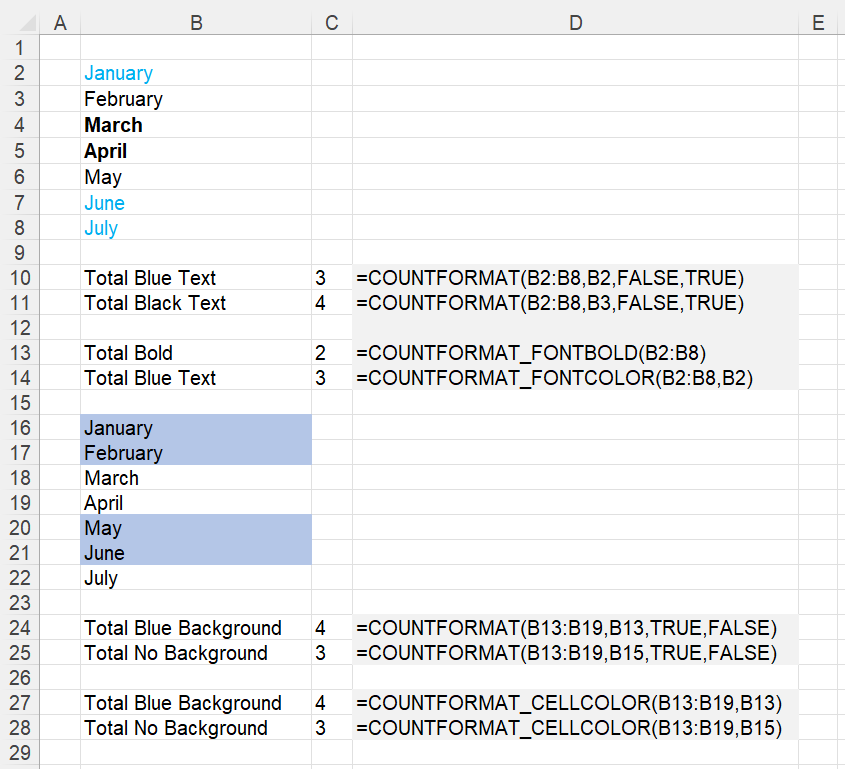# COUNTFORMAT

4 Functions that allow you to count different types of formatting.
Need a function that can detect any cell formatting (not a specific colour)

### Remarks

* For instructions on how to add a function to a workbook refer to the page under Inserting Functions
* The equivalent JavaScript function is COUNTFORMAT

### COUNTFORMAT

Returns the number of cells that have been formatted with multiple attributes.
This function will not update automatically every time a cell format changes although pressing F9 will work.

`Option Explicit 'rgeValues - The range of values'rgeExampleCell - The cell whose format you want to count'bCheckBackGround - Whether you want to check the background formatting'bCheckFontColor - Whether you want to check the text formattingPublic Function COUNTFORMAT( _             ByVal rgeValues As Range, _             ByVal rgeExampleCell As Range, _    Optional ByVal bCheckBackGround As Boolean = True, _    Optional ByVal bCheckFontColor As Boolean = False, _    Optional ByVal bCheckFontBold As Boolean = False) As Integer Dim objcell As Range Dim itotalcells As Integer Dim bbackground_check As Boolean Dim bfontcolour_check As Boolean Dim bfontbold_check As Boolean    itotalcells = 0    For Each objcell In rgeValues       bbackground_check = True       bfontcolour_check = True       bfontbold_check = True          If (bCheckBackGround = True) Then          If objcell.Interior.ColorIndex <> rgeExampleCell.Interior.ColorIndex Then             bbackground_check = False          End If       End If       If (bCheckFontColor = True) Then          If objcell.Font.ColorIndex <> rgeExampleCell.Font.ColorIndex Then             bfontcolour_check = False          End If       End If       If (bCheckFontBold = True) Then          If (objcell.Font.Bold = False) Then             bfontbold_check = False          End If       End If          If (bbackground_check = True) And (bfontcolour_check = True) And (bfontbold_check = True) Then          itotalcells = itotalcells + 1       End If       Next objcell       COUNTFORMAT = itotalcells End Function `### COUNTFORMAT_CELLCOLOR

Returns the number of cells that have been formatted with a particular colour.

`'rgeSearchCells - The range of cells you want to check'rgeExampleCell - The cell with the colour you want to countPublic Function COUNTFORMAT_CELLCOLOR( _          ByVal rgeColouredCells As Range, _          ByVal rgeExampleCell As Range) As Long Dim ltotal As Long Dim rgecell As Range Dim lcellcolour As Long    lcellcolour = rgeExampleCell.Interior.ColorIndex    For Each rgecell in rgeColouredCells       If rgecell.Interior.ColorIndex = lcellcolour Then          ltotal = ltotal + 1       End If    Next rgecell    COUNTFORMAT_CELLCOLOR = ltotal End Function `

### COUNTFORMAT_FONTBOLD

Returns the number of cells that have been formatted in bold.

`'rgeSearchCells - The range of cells you want to checkPublic Function COUNTFORMAT_FONTBOLD( _          ByVal rgeSearchCells As Range) As Long     Dim ltotal As Long Dim rgecell As Range    For Each rgecell In rgeSearchCells       If (rgecell.Font.Bold = True) Then          ltotal = ltotal + 1       End If    Next rgecell            COUNTFORMAT_FONTBOLD = ltotal End Function `

### COUNTFORMAT_FONTCOLOR

Returns the number of cells that have been formatted with a particular font colour.

`'rgeSearchCells - The range of cells you want to check'rgeExampleCell - The cell with the font colour you want to countPublic Function COUNTFORMAT_FONTCOLOR( _          ByVal rgeSearchCells As Range, _          ByVal rgeExampleCell As Range) As Long     Dim ltotal As Long Dim lfontcolor As Long Dim rgecell As Range    lfontcolor = rgeExampleCell.Font.Color    For Each rgecell In rgeSearchCells       If (rgecell.Font.Color = lfontcolor) Then          ltotal = ltotal + 1       End If    Next rgecell            COUNTFORMAT_FONTCOLOR = ltotal End Function `